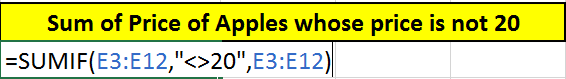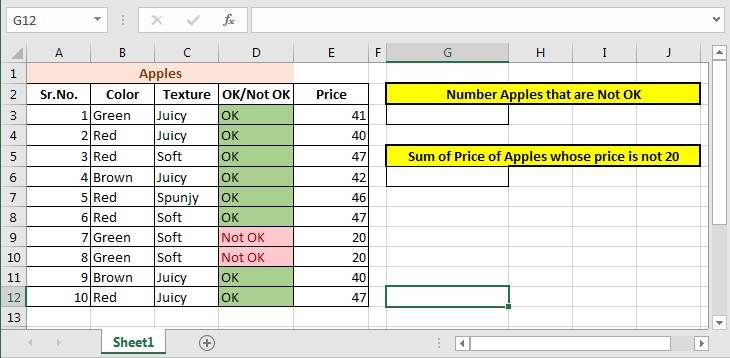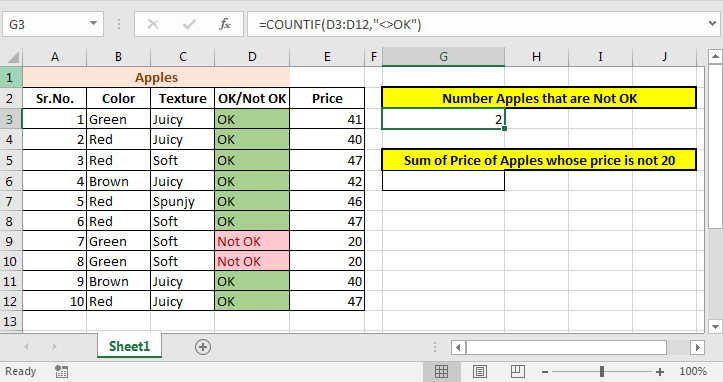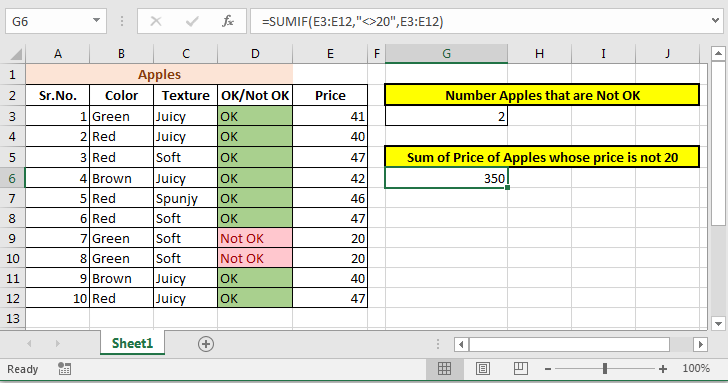# How to use the Operations If Not Equal To in Excel

Excel uses <> sign as not equal to operator. Put “<>” between two expressions to use it. This (<>) operator excludes any matching value from calculation.### Count If Not Equal To

#### Generic Formula

=COUNTIF(range,”<>value”)
=COUNTIF(range,”<>& Cell Reference)

Example:

So we have this data of Apples.We want to count apples that are Not OK using not equal to (<>) sign.

Write this formula in Cell G3:

=COUNTIF(D3:D12,"<>OK")

This COUNTIF exclude all OK values from range D3:D12 and counts remaining values.### Sum If Not Equal To

#### Generic Formula

=SUMIF(sum_range,”<>value”, sum_range)
=SUMIF(sum_range,”<>& Cell reference, sum_range)

Now lets sum the prices whose value is not equal to 20.

Write SUMIF formula in Cell G6:

=SUMIF(E3:E12,"<>20",E3:E12)

This The SUMIF function will exclude all values that are equal to 20 in range D3:D12 and sums remaining values.So yeah guys, this is where most people get stuck but its easy to do. You just didn’t know about Not Equal To operator in Excel. Now you do.

Related Articles:

How to use the Excel NOT function in Excel

How to Calculate Only If Cell is Not Blank in Excel

IF not this or that in Microsoft Excel

How to Sum if cell is not equal to value in Excel

How to Count cells that do not contain value in Excel

Popular Articles:

50 Excel Shortcuts to Increase Your Productivity

How to use the VLOOKUP Function in Excel

How to use the COUNTIF function in Excel

How to use the SUMIF Function in Excel

Terms and Conditions of use

The applications/code on this site are distributed as is and without warranties or liability. In no event shall the owner of the copyrights, or the authors of the applications/code be liable for any loss of profit, any problems or any damage resulting from the use or evaluation of the applications/code.